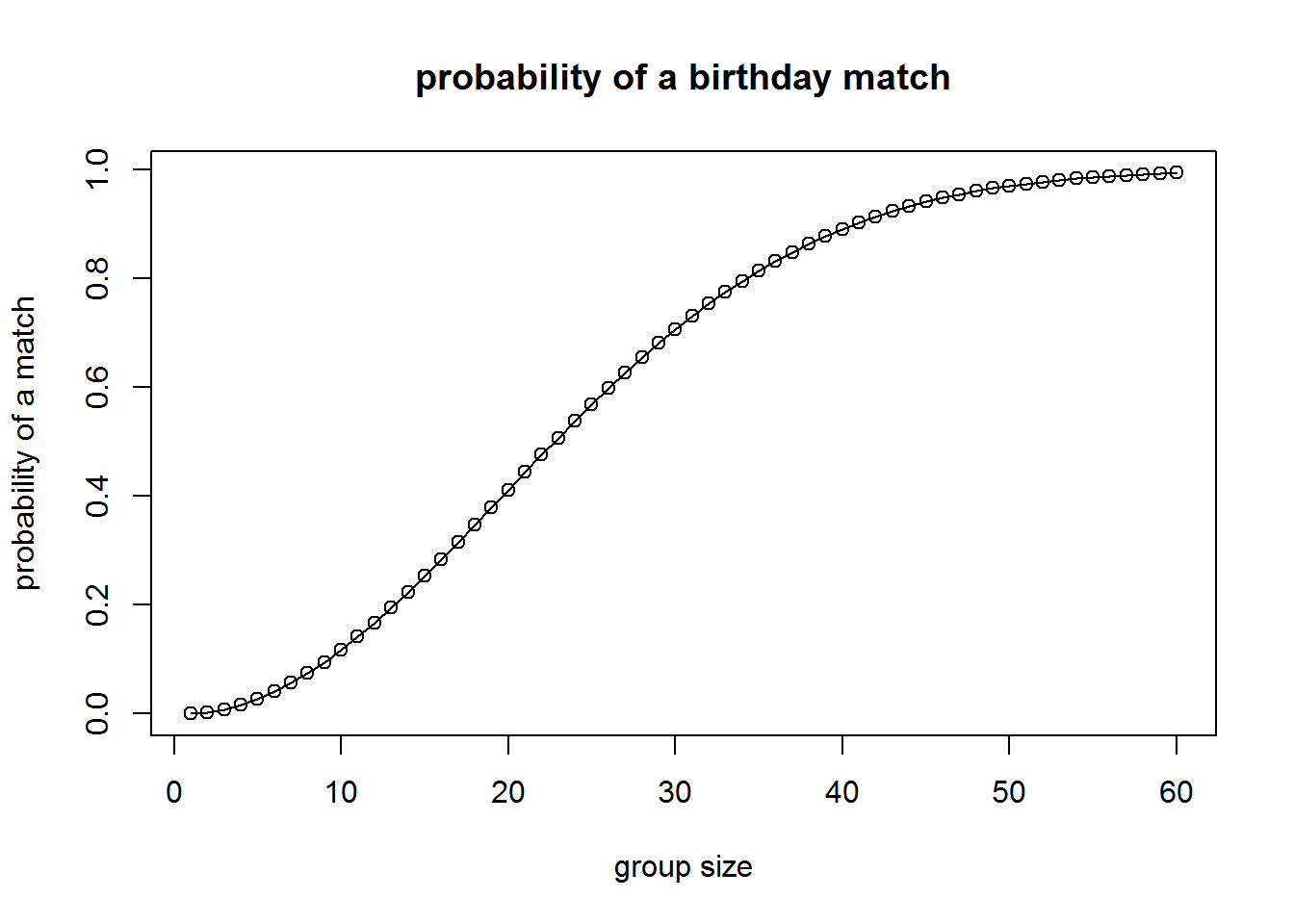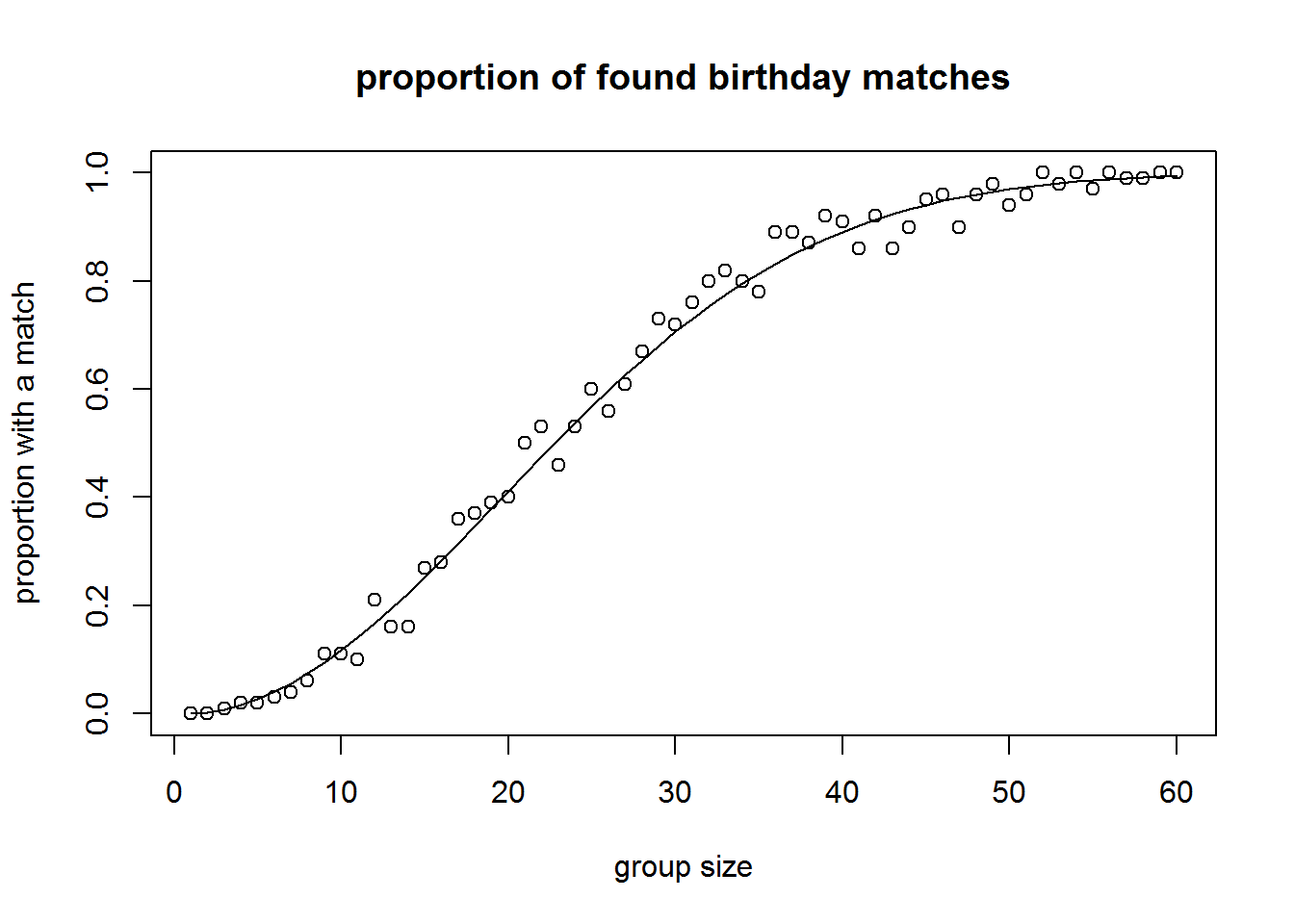## Introduction

The Birthday Problem, as frequently happens in questions of probability, is easy to state and understand, yet leads to an (initially) suprising solution.

In a group of n people, how likely is it to find two or more people sharing the same birthday?

Many people are surprised to find out that in a group of 23 people, there is just over a fifty percent chance of finding a birthday match, and in a group of 60 people, you are almost certain to find two or more people sharing a birthday.

## Direct Calculation

If $$B_n$$ is the event that we find two or more people in a group of $$n$$ that share a birthday, then $$\neg B_n$$, is the probability that there are no birthday matches in a group of $$n$$ people, and the probability of this event is a bit easier to calculate.

In a group of one, $$n = 1$$, there is no chance to find two or more people that share a birthday. In a group of two, the probabability of not sharing a birthday is $$p(\neg B_2) = \frac{364}{365}$$. For a group of three, we have $$p(\neg B_2) = \frac{364}{365} \times \frac{363}{365}$$, and in general:

$p(\neg B_n) = \prod_{i=1}^n \frac{366-i}{365}$

We can set up a data frame BCalc in R to calculate this directly.

group_size <- 60
group <- 1:group_size
BCalc <- data.frame(group)
BCalc$noMatch = 1; for (i in 2:group_size) { BCalc$noMatch[i] <- BCalc$noMatch[i-1]*(366-i)/365 } BCalc$match <- 1 - BCalc$noMatch Plotting this relationship between the probability of a birthday match and the size of the group involved gives a characteristic sigmoid or logistic curve. plot(BCalc$group, BCalc$match,main='probability of a birthday match', xlab = 'group size', ylab='probability of a match') lines(BCalc$group, BCalc$match)This gives the somewhat surprising result that, in a group of 23 people there is over a fifity percent chance of finding two people with the same birthday. BCalc$match
##  0.5072972

By the time you have sixty people together, it is almost a sure thing to find a match.

BSim$foundMatch[current_index] <- as.logical(anyDuplicated(t)) current_index <- current_index +1 } } ## Exploring the Simulation For each group size, we can find the proportion of groups that contained a match, and plot the results in comparison to the expected curve from the BCalc data frame. tbl <- table(BSim$groupSize,BSim$foundMatch) mtrx <- as.matrix(prop.table(tbl,1)[,2]) plot(1:group_size, mtrx[,1],main='proportion of found birthday matches', xlab = 'group size', ylab='proportion with a match') lines(BCalc$group, BCalc\$match)